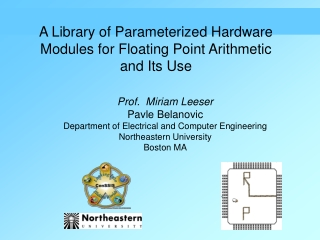DownloadDownload PresentationA Library of Parameterized Hardware Modules for Floating Point Arithmetic and Its Use

# A Library of Parameterized Hardware Modules for Floating Point Arithmetic and Its Use

Télécharger la présentation## A Library of Parameterized Hardware Modules for Floating Point Arithmetic and Its Use

- - - - - - - - - - - - - - - - - - - - - - - - - - - E N D - - - - - - - - - - - - - - - - - - - - - - - - - - -
##### Presentation Transcript

1. A Library of Parameterized Hardware Modules for Floating Point Arithmetic and Its Use Prof. Miriam LeeserPavle Belanovic Department of Electrical and Computer Engineering Northeastern University Boston MA

2. Outline • Introduction and motivation • Library of hardware modules for variable precision floating point • Application: K-means algorithm using variable precision floating point library • Conclusions

3. Accelerating Algorithms • Reconfigurable hardware used to accelerate image and signal processing algorithms • Exploit parallelism for speedup • Customize design to fit the particular task • signals in fixed or floating-point format • area, power vs. range, precision trade-offs

4. narrower wider Fewer hardware resources Better range, precision Signal Bitwidth More parallelism Less power dissipation Format Design Trade-offs Goal: use optimal format For every value

5. IEEE single precision 12-bit floating point format 31 adders 113 adders Xilinx XCV1000 FPGA OR OR 13 multipliers 85 multipliers Area Gains Using Reduced Precision

6. sign exponent fraction / mantissa MSB LSB General Floating-Point Format (-1)s * 1.f * 2e-BIAS

7. 1 8 23 exp (e) s fraction (f) 32 IEEE Floating Point Format • BIAS depends on number of exponent bits • 127 in IEEE single precision format • Implied 1 in mantissa not stored (-1)s * 1.f * 2e-BIAS

8. format control operators conversion Library of Parameterized Modules • Total of seven parameterized hardware modules for arbitrary precision floating-point arithmetic

9. Highlights • Completely general floating-point format • All IEEE formats are a subset • All previously published non-IEEE formats are a subset • Abstract normalization from other operations • Rounding to zero or nearest • Pipelining signals • Some error handling

10. Assembly of Modules 2  denorm + 1  fp_add + 1  rnd_norm = 1  IEEE single precision adder

11. Denormalization • “Unpack” input number: insert implied digit • If input is value zero, insert ‘0’ Otherwise, insert ‘1’ • Output 1 bit wider than input • Latency = 0

12. Rounding and Normalizing • Returns input to normalized format • Designed to follow arithmetic operation(s)

14. Multiplication

15. Fixed to Floating-Point

16. Floating to Fixed-Point

17. Implementation Experiments • Designs specified in VHDL • Mapped to Xilinx Virtex FPGA • Wildstar reconfigurable computing engine by Annapolis Micro Systems Inc. • PCI Interface to host processor • 3 Xilinx XCV1000 FPGAs • total of 3 million system gates • 40 Mbytes of SRAM • 1.6 Gbytes/sec I/O bandwidth • 6.4 Gbytes/sec memory bandwidth • clock rates to 100MHz

18. Synthesis Results

19. Synthesis Results

20. K-means Algorithm Image spectral data Pixel Xij Clustered image mag (12 bits) class 0 class 1 class 2 class 3 j = 0 to J 0 1 2 3 4 class 4 spectral component ‘k’ k = 0 to K Every pixel Xij is assigned a class cj i = 0 to I • Each cluster has a center: • mean value of pixels in that cluster • Each pixel is in the cluster whose center it is closest to • requires a distance metric • Algorithm is iterative

21. Hardware Implementation of k-means Clustering 8x Cluster Distance Measure Pi,0 dist Cj,0 + Pi,1 dist Cj,1 + Pi,2 min dist Cj,2 + min Pi,3 Cluster Number dist + Cj,3 min min . . . . . . 10 input channels . . . . . + min min + min 290 16 bit operations

22. K-means Clustering Algorithm Purely fixed-point Hybrid fixed and floating-point

23. Structure of the K-means Circuit

24. Hybrid Datapath

25. Results of Processing Purely fixed-point Hybrid fixed and floating-point

26. Synthesis Results

27. Conclusions • Library of fully parameterized hardware modules for floating-point arithmetic available • Ability to form arithmetic pipelines in custom floating-point formats demonstrated • Future work • More floating point modules (ACC, MAC, DIV ...) • More applications • Automation of design process using the library • Automatically choose best format for each variable and operation